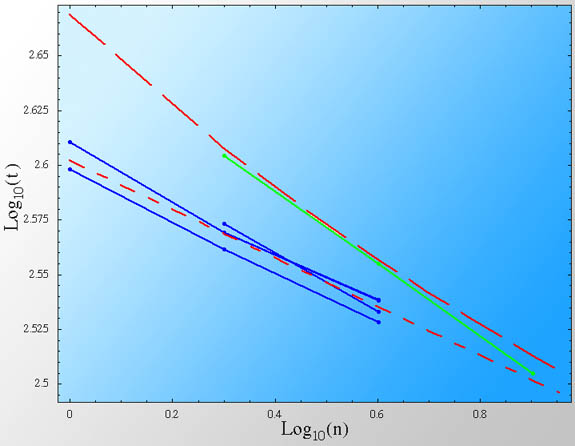Row Row Row your Boat - At which maximum speed?The maximum speed of a rower boat is a classic example of the beauty of dimensional analysis. It was originally derived by McMahon (1971). Here it is brought again for the convenience of the Internet audience, together with updated rowing record data.

First, some assumptions (some are better than others).
1. Boats with different rowers are geometrically similar to each other.
2. The submerged volume of a boat, per rower, is the same and equal to $V_0$
3. The power supplied by a rower is always the same and equal to $P_{0}$
Second, we need to understand which physical processes govern the maximum speed of the rowing boats. The dominant drag force is that of friction with the water. Note that if the boat was larger or the speed faster, the typical Froude number would have been large. In such a case, the drag would have been governed by the generation of surface gravity waves (or "waves" for short). For a typical rowing boat, the Froude number is small and hence the effect of gravity waves is negligible.

Since the motion of the water is turbulent (there are vortices behind the boat), the frictional drag force is given by: $$F \sim\rho v^{2} \ell^2.$$ (This can be seen from dimensional analysis, the only "force" we can construct from the density of water $\rho$, the speed of the boat $v$ and its dimension $\ell$ is $\rho v^2 \ell^2$).

The power lost to drag is therefore: $$P_{drag} = F v \sim \rho v^3 \ell^{2}.$$ Since we assume geometric similarity, the size of the boat $\ell$ will be proportional to the submerged depth, which itself should be proportional to the cubic root of the submerged volume. Thus, $\ell \sim V^{1/3} \approx {(N V_0)}^{1/3}$.

On the other hand, the total power supplied by the rowers, $P_{rowers}$, should be proportional to the number of rowers $N$, each supplying a power of $P_0$

At steady state, the power of the rowers should be the same as the power lost to drag. We thus find:
$$P_{rowers} \approx P_{drag} ~~\Rightarrow ~~ N P_0 \sim \rho v^3 N^{2/3} {V_0}^{2/3} ,$$
or: $$v\sim {N^{1/9} P_0^{1/3} \over \rho^{1/3} V_0^{2/9}}.$$ In other words, the speed of an $N$ rower boat scales as $N^{1/9}$. The time it would take them to finish a race will therefore scale as $t \propto N^{-1/9}$.

Different type boats will of course have a different prefactor, since $V_0$ and $C_D$ (the drag coefficient, which is the prefactor in the drag equation) are somewhat different. But for a given type, we should see this scaling behavior.

What happens if there is a coxswain? In such a case, there is an extra person who does nothing (well, from a physical point of view), except add weight and volume. This implies that now, the power supplied by the $N$ rowers is the same, but the drag they need to overcome is larger:
$$N P_0 \sim \rho v^3 (N+1)^{2/3} {V_0}^{2/3} ,$$
or:
$$v \sim {N^{1/3} (N+1)^{-2/9} P_0^{1/3} \over \rho^{1/3} V_0^{2/9}} .$$
Both results, with and without a coxswain can be seen in the graph.

World RecordsWorld Record for different classes of rower boats over 2000m races, as a function of number of rowers (as updated on Sep. 2004). Note that it is a Log-Log plot such that a power-law would appear as a straight line. The blue dots (and lines) are Men classes: Mnx, Mn-, LMnx, LMn-, which are rower boats without coxswains, while the green line is Mn+, i.e., with coxswains. n stands for the number of rowers. The short red dashed line is the prediction (up to a normalization) of t ~ N-1/9. The long red dashed line is the prediction when a coxswain is present (with the same normalization as without). The two lines asymptotically approach each other for large N.

Evidently from the graph, the predicted scaling of $t\propto N^{-1/9}$ for rower boats without coxswains, and $t \propto N^{-1/3} (N+1)^{2/9}$ with coxswains very nicely explains the observed world records. In fact, all are consistent with the prediction to within about 1.5 seconds or less!

Of course, given that we deliberately forgot all the prefactors (such as $C_D$), the absolute normalization can be estimated, up to an order of manitude. If we plug in typical values: $P_0 \approx 500 W$, $V_0 \approx 72 kg/ \rho_w$ and $\rho_w = 1000~kg/m^3$, we find that the normalization (i.e., the time for a one man rowing boat, over a D=2000m race) is:
$$t_1 = {D \over v} \sim { 2000m \times (1000 kg/m^3)^{1/3} (72/1000 m^3)^{2/9} \over (500W)^{1/3}} \approx 1400 s,$$
In reality, it is of course around 400s. The discrepancy is mostly because $C_D$, multiplying the drag force, is actually small, only a few percent (e.g., about 2%). Since $t \propto C_D^{1/3}$, a better estimate would be $t \sim 1400 s\times C_D^{1/3} \sim 400 s$ as observed.

Another interesting point. if one compares the world records for men and women, of the same class boats, it is evident that women records are about 8% longer. Since the time is proportional to $P^{-1/3}$, it implies that the best men rowers can deliver power from their muscles at a rate which about 8%*3 ~ 25% higher than the best women rowers. Men are about 25% stronger than women, but of course less pretty and often less smart as well.

Bibliography:
McMahon, T.A. 1971. "Rowing: A similarity analysis". Science 173:349.
Peterson, I, 1999. "Row Your Boat", science news online.

Blogroll

Sensible Climate Physics and more Other